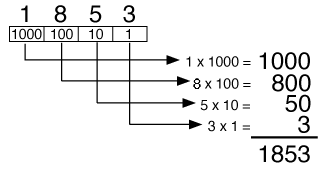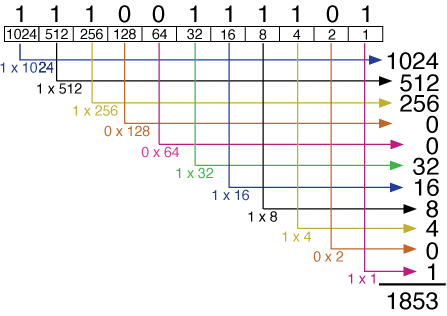# Computer Concepts and Terminology

 Home Intro History Types Software Binary Hardware Input/Output Processors Memory Storage Ports Net/Telecom Questions## Binary Representation

Your personal computer is a type of digital electronic computer. It is called digital because all of the information inside it is represented and manipulated as numbers (the original meaning of “digit” is “finger,” and since people often count using their fingers, the term digit also came to to be applied to numbers). All of the numbers in a spreadsheet, all of the text characters in a Word document, all of the pictures and sounds stored in a computer, are ALL represented as numbers.

The number system that you use is base 10 (since people have 10 fingers, this works out well for them). When you write the number 1853, for example it means:Each digit (0-9) within a base 10 number is multiplied by the power of ten corresponding to its position. Notice that each digit place has 10 times the value of the digit place to the right of it. But you knew all this, of course.

### Binary Numbers

But what of the poor computer, which has no fingers to count on? Base 10 is not convenient for a fingerless computer to use. What computers DO have, are electrical circuits, which are either on or off. Just two states to work with. So the natural number system for use in an electronic computer is base 2 (called the binary number system). Unlike you who have ten digits to calculate with (0, 1, 2, 3, 4, 5, 6, 7, 8, 9), the computer has only two digits (0 and 1) with which it must do everything. So, in a computer’s memory, a tiny transistor that is on (conducting a current) might represent a 1, while a transistor that is off would represent a 0 (zero).

The binary number 11100111101, for example, means:Ah! So they are the same number!

1853 (base 10)  =  11100111101 (base 2)

Notice that each binary digit position in the base 2 number has 2 times the value of the binary digit position to the right of it (since this is base 2; remember how base 10 worked).

It gets cumbersome saying “binary digit” all the time, so the shorter term “bit” was invented. A bit is one binary digit. A bit can hold either a 1 or a 0 (zero). A string of bits can hold larger numbers (just as you use strings of base 10 digits to represent numbers larger than 9).

 Binary Representation of Numbers Base 10 Base 2 0 00000000 1 00000001 2 00000010 3 00000011 4 00000100 5 00000101 ... ... 65 01000001 66 01000010 67 01000011 ... ... 254 11111110 255 11111111

A particularly handy size chunk of computer memory happens to be 8 bits long. This size chunk of memory can be used to represent any number from zero (00000000) to 255 (11111111). Why does 11111111 (base 2) equal 255 (base 10)? Because it means:

1 x 128  +  1 x 64  +  1 x 32  +  1 x 16  +
1 x 8  +  1 x 4  +  1 x 2  +  1 x 1  =  255

And why is this a handy size chunk of memory? Because if we want to represent all of the characters of the English alphabet, 8 digits is the first power of 2 that gives you enough possibilities to do this (a 4-bit long chunk can only hold numbers from zero to 7...not enough).

We have a special name for a chunk of memory that is 8 bits long: it is called a byte. This is the basic unit we use to measure computer memory size. (A chunk of memory 4 bits long is called a “nybble,” but you don't need to know that for the test.)

Text characters are represented in computer memory as numbers. How? You need a scheme for equating letters to numbers. The system used is called the ASCII code (American Standard Code for Information Interchange). The capital letter A is represented by the number 65 in the ASCII code (65 is 01000001 in binary). The first 65 ASCII codes (0 through 64) are used for an assortment of Control characters and special characters, so capital A ended up at 65. Capital B is 66 (01000010) and so on.

 ASCII Representation of Characters (just a sample) Character Base 10 Base 2 (return) 13 00001101 (space) 32 00100000 ! 33 00100001 1 49 00110001 2 50 00110010 @ 64 01000000 A 65 01000001 B 66 01000010 C 67 01000011 a 97 01100001 b 98 01100010 c 99 01100011 (delete) 127 01111111

How does the computer know whether the 01000001 in a byte of memory is the number 65 or the letter A? Because an application program keeps track of what it put where in memory, so MS Word knows that a given byte where it has stored text contains numbers that represent letters.

For foreign alphabets that contain many more letters than English (such as Japanese Kanji) a newer extension of the the ASCII scheme called Unicode is now used (it uses two bytes to hold each letter; two bytes give 65,535 different values to represent characters).

Pictures are also represented as numbers in the computer. If you look closely at your display screen, you can see that the image on it is made up of lots of little spots, called picture elements (which is more commonly shortened to pixel). Each pixel in a screen image might be represented by three bytes in the computer; the numbers in the bytes tell the display how much red, blue, and green light should be mixed together to make the color of the pixel (three bytes can represent millions of possible colors for each pixel).

The programs that a computer executes are also stored as numbers. Each number in this case represents an instruction for the microprocessor (each operation the processor can perform, such as “fetch a number into a register” and “add the contents of two resister together,” are represented by unique binary codes).

### Kilobytes, Megabytes, Gigabytes, etc.

Memory capacity and data storage capacity for computers are measured in bytes. File sizes are also measure in bytes (one byte is 8 bits, remember). However, a byte is small (it can hold only one character) so we use larger units:

A kilobyte (KB) is approximately 1,000 bytes. But it is NOT exactly 1,000 bytes; it is 1,024 bytes. Why a strange number like 1,024? Because 1,024 is exactly 10000000000 in binary; a nice multiple of two is very handy for the computer. So remember: When the computer tells you that your file takes up 40 kilobytes, it is actually using 40,960 bytes (not 40,000). But you can think of a kilobyte as “roughly 1,000 bytes,” which is how it got its name. This web page file is approximately 20 KB in size.

Similarly, you can think of a megabyte (MB) as approximately a million bytes, but it is precisely 1,048,576 bytes (1,024 x 1,024). The MS Word application takes up about 13 MB on the computer’s hard disk (depending on version). A typical personal computer may have 512 MB of memory.

A gigabyte (GB) is approximately one billion bytes (1,073,741,824 exactly). The root word for the giga is the same one our word giant comes from, so gigabyte should technically be pronounced with a soft g—but the pronunciation with either hard or soft g is acceptable. The storage capacity of a typical hard disk is measures in the tens or hundreds of GB.

In case you are wondering, a trillion bytes is a terabyte, but PC capacities haven’t gotten into that range yet.

Side Note: Just to make things more confusing, many manufacturers state the capacities of their hard disks and other devices in terms of kilobytes that are defined to be exactly 1,000 bytes. This has the advantage (for them) of making their products seem 2.4% bigger. For purposes of this class, we will use the definition of a kilobyte = 1,024 bytes.